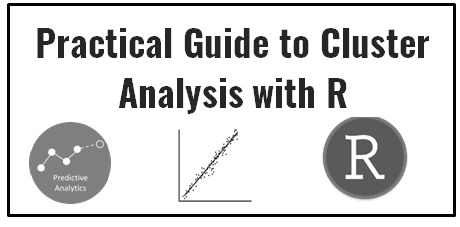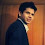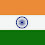# Clustering in R

This tutorial covers various clustering techniques in R. R supports various functions and packages to perform cluster analysis. In this article, we include some of the common problems encountered while executing clustering in R.

Cluster Analysis
Finding similarities between data on the basis of the characteristics found in the data and grouping similar data objects into clusters. It is an unsupervised learning technique (No dependent variable).

Examples of Clustering Applications

Marketing: Help marketers discover distinct groups in their customer bases, and then use this knowledge to develop targeted marketing programs.

Insurance: Identifying groups of motor insurance policy holders with some interesting characteristics.

Games: Identify player groups on the basis of their age groups, location and types of games they have shown interest in the past.

Internet: Clustering webpages based on their content.Practical Guide to Cluster Analysis with R

Quality of Clustering

A good clustering method produces high quality clusters with minimum within-cluster distance (high similarity) and maximum inter-class distance (low similarity).

Ways to measure Distance

For Continuous Variables

Manhattan distance: |x2-x1| + |y2-y1|

For Categorical Variables

1. Hamming Distance

It can be calculated by sum of different or dissimilar digits / categories.

If the categorical variable is binary, we can straight away calculate Hamming distance. If the number of categories are more than two, we need to convert these categories into a set of dummy binary variables. Let' say we have two variables - Sex and Status. The variable 'sex' has two levels - Male (0) and Female (1) whereas the variable 'status' has 3 levels - High, Medium, Low.

1. Dave - (Male, High)
2. Mat - (Male, Low)
3. Jenny - (Female, High)

Let's create 3 binary variables for status and set the coordinates.
Dave = (0, (1, 0, 0))
Mat  = (0, (0, 0, 1))
Jenny = (1, (1, 0, 0))
To compute the distance between object, we need to calculate it for each original variable. Let's calculate Hamming Distance ( Dissimilar Categories).

Let's say :

a = number of variables that positive (1) for both individuals.
b = number of variables that positive (1) for one individual and negative (0) for other individual.
c = number of variables that negative (0) for one individual and positive (1) for other individual.
d = number of variables that negative (0) for both individuals.
Hamming Distance = (b + c)
Distance (Dave, Mat) is  (0, 2) , overall distance  is 0+2 = 2
Distance (Dave, Jenny) is (1, 0) , overall distance is 1+0 = 1
Distance (Mat, Jenny) is (1, 2) , overall distance is 1+2 = 3

2. Simple Matching Distance (SMD)

It is the ratio of number of unmatched dummy variables to total number of dummy variables. The distance is calculated based on the original variables. First we need to calculate distance based on dummy variables.
SMD = (b + c) / (a + b + c + d)
Distance (Dave, Mat) is  (0, 2/3) , average distance is (0+(2/3))/2 = 1/3.
Distance (Dave, Jenny) is (1, 0) ,  average distance is (1+0)/2 = 1/2
Distance (Mat, Jenny) is (1, 2/3) , average distance is (1+2/3)/2 = 5/6

Note - Distance (Dave, Mat) is  (0, 2/3) can be read as  :

1. 0/1 which is [number of mismatch dummy variable (which is 0) / Number of dummy variable for Gender i.e. 1]
2. 2/3 == [Number of mismatch dummy variables in Status / Number of dummy variables for Status]

3. Jaccard Measure

It's almost same as SMD as explained above. The only difference is Jaccard Index does not include [number of dummies 0 for both] in denominator.
J = (b + c) / (a + b + c)
Jaccard Measure is considered more robust than Simple Matching distance method as it ignores zero for both dummy variables.

4. Dice Coefficient
D = 2a / (2a + b + c)
It is more appropriate for dummy variables. For dummy variables, it is equivalent to cosine.

Data Preparation
2. Standardize Continuous Variables
3. Remove outliers
4. Variable Type : Continuous or binary variable
5. Check Multicollinearity

Types of Clustering
1. K-means Clustering (Flat Clustering)
2. Hierarchical clustering (Agglomerative clustering)

Detailed Theoretical Explanation - How Cluster Analysis works

Assess clustering tendency (clusterability)

It is important to assess cluster tendency (i.e. to determine whether data contain meaningful clusters) before running any clustering algorithms. In unsupervised learning, the clustering methods returns clusters even if the data does not contain any meaninful cluster pattern.

Hopkins statistic is used to assess the clustering tendency of a dataset by measuring the probability that a given dataset is generated by a uniform data distribution (i.e. no meaningful clusters).

The null and the alternative hypotheses are defined as follow:
1. Null hypothesis: the dataset is uniformly distributed (i.e., no meaningful clusters)
2. Alternative hypothesis: the dataset is not uniformly distributed (i.e., contains meaningful clusters)
If the value of Hopkins statistic is close to 0,it means that the data is highly clusterable. If the value is close to 0.5, that means the data contains no meaningful clusters.

Determine the optimal number of clusters

In R, there is a package called "NbClust" that provides 30 indices to determine the optimal number of clusters. The 2 important methods out of 30 methods are as follows -
1. Look for a bend or elbow in the sum of squared error (SSE) scree plot. The location of the elbow in the plot suggests a suitable number of clusters for the kmeans.
2. Silhouette analysis measures how well an observation is clustered and it estimates the average distance between clusters. The silhouette plot displays a measure of how close each point in one cluster is to points in the neighboring clusters. A higher silhouette width is preferred to determine the optimal number of clusters. Observations with a negative width are probably placed in the wrong cluster.

Important Steps : Cluster Analysis
1. Select important variables for analysis
2. Check and remove outliers
3. Standardize variables
4. Assess clusterability
5. Select the right clustering algorithm and optimal no. of clusters - Internal Validation (clValid Package)
6. External Validation (if true label available)

Clustering for Mixed Data

K-mean clustering works only for numeric (continuous) variables. For mixed data (both numeric and categorical variables), we can use k-prototypes which is basically combining k-means and k-modes clustering algorithms.

For numeric variables, it runs euclidean distance. For categorical variables, k-modes use Simple Matching distance which is explained above. In k-modes, modes act as centroids (i.e. cluster center). It minimizes the sum of distances between each object in the cluster and centroid.

K-prototype algorithm works as follows -

1. Select k initial prototypes from a data set X, one for each cluster. Here, prototypes are cluster centers - means / modes. In k-modes clustering, the cluster centers are represented by the vectors of modes of categorical attributes. In statistics, the mode of a set of values is the most frequent occurring value. There can be more than one mode in a set of values. If a data set has m categorical attributes, the mode vector Z consists of m categorical values, each being the mode of an attribute.
Cluster prototypes are computed as cluster means for numeric variables and modes for categorical variables. Randomly select k objects as the initial cluster centers.
2. Calculate the distances between each object and the cluster prototype; assign the object to the cluster whose center has the shortest distance to the object; repeat this step until all objects are assigned to clusters. Update the prototype of the cluster after each allocation.
dist(x, y) = euclid(numeric_variables) + λ* simple matching(categorical_variables)
Here λ : If it is greater than 0 to trade off between Euclidean distance of numeric variables and simple matching coefficient between categorical variables.

3. After all objects have been allocated to a cluster, retest the similarity of objects against the current prototypes. If an object is found such that its nearest prototype belongs to another cluster rather than its current one, reallocate the object to that cluster and update the prototypes of both clusters.

4. Repeat step 3 until no object has changed clusters.

One more approach for handling Mixed Data

Step 1. Calculate Gower Distance which runs Manhattan distance for continuous variables, manhattan with some adjustments for ordinal variables, and dice coefficient for nominal variables after converting them to binary variables.

Step 2. Run Hierarchical Clustering / PAM (partitioning around medoids) algorithm using the above distance matrix. PAM algorithm works similar to k-means algorithm. The steps are as follows -
1. Choose k random entities to become the medoids
2. Assign every entity to its closest medoid (using our custom distance matrix in this case)
3. For each cluster, identify the observation that would yield the lowest average distance if it were to be re-assigned as the medoid. If so, make this observation the new medoid.
4. If at least one medoid has changed, return to step 2. Otherwise, end the algorithm.

3. Select the number of clusters using silhouette width method.

R Code : Cluster Analysis (Numeric Variables)

Note : We can automate selecting the best clustering algorithm and optimal number of clusters with clValid Package.
data<-iris[,-c(5)]

# To standarize the variables
data = scale(data)

# Assessing cluster tendency
if(!require(clustertend)) install.packages("clustertend")
library(clustertend)
# Compute Hopkins statistic for the dataset
set.seed(123)
hopkins(data, n = nrow(data)-1)
#Since the H value = 0.1815 which is far below the threshold 0.5, it is highly clusterable

###########################################################################
####################### K Means clustering ################################
###########################################################################

# K-mean - Determining optimal number of clusters
# NbClust Package : 30 indices to determine the number of clusters in a dataset
# If index = 'all' - run 30 indices to determine the optimal no. of clusters
# If index = "silhouette" - It is a measure to estimate the dissimilarity between clusters.
# A higher silhouette width is preferred to determine the optimal number of clusters

if(!require(NbClust)) install.packages("NbClust")
nb <- NbClust(data,  distance = "euclidean", min.nc=2, max.nc=15, method = "kmeans",
index = "silhouette")
nb\$All.index
nb\$Best.nc

#Method II : Same Silhouette Width analysis with fpc package
library(fpc)
pamkClus <- pamk(data, krange = 2:15, criterion="multiasw", ns=2, critout=TRUE)
pamkClus\$nc
cat("number of clusters estimated by optimum average silhouette width:", pamkClus\$nc, "\n")

#Method III : Scree plot to determine the number of clusters
wss <- (nrow(data)-1)*sum(apply(data,2,var))
for (i in 2:15) {
wss[i] <- sum(kmeans(data,centers=i)\$withinss)
}
plot(1:15, wss, type="b", xlab="Number of Clusters",ylab="Within groups sum of squares")

# K-Means Cluster Analysis
fit <- kmeans(data,pamkClus\$nc)

# get cluster means
aggregate(data,by=list(fit\$cluster),FUN=mean)

# append cluster assignment
data <- data.frame(data, clusterid=fit\$cluster)

###########################################################################
####################### Hierarchical clustering############################
###########################################################################

# Hierarchical clustering - Determining optimal number of clusters
library(NbClust)
res<-NbClust(data, diss=NULL, distance = "euclidean", min.nc=2, max.nc=6,
method = "ward.D2", index = "kl")
res\$All.index
res\$Best.nc

# Ward Hierarchical Clustering
d <- dist(data, method = "euclidean")
fit <- hclust(d, method="ward.D2")
plot(fit) # display dendogram

# cluster assignment (members)
groups <- cutree(fit, k=2)
data = cbind(data,groups)

# draw dendogram with red borders around the 2 clusters
rect.hclust(fit, k=2, border="red")
Next Step : Validate Cluster Analysis

R : Cluster Analysis for Mixed Data

Make sure you convert categorical variables to factor before running kproto function.
```# Install and load desired package
install.packages("clustMixType")
library(clustMixType)

# Apply k prototypes
a <- lambdaest(mydata)
res <- kproto(mydata, k= 2, lambda = a)
clprofiles(res, mydata)```
The lambdaest function compares variances to specify lambda for k prototypes clustering.

Predict Clusters on New Data

Idea is to extract cluster centers from k-mean clustering and then compute euclidean distance between each observation of  new data and each cluster center and then find closest cluster to each observation wherein minimum distance.
# Training Data
set.seed(144)
x <- rbind(matrix(rnorm(100, sd = 0.3), ncol = 2),
matrix(rnorm(100, mean = 1, sd = 0.3), ncol = 2))
colnames(x) <- c("x", "y")

# New Data
x_new <- rbind(matrix(rnorm(10, sd = 0.3), ncol = 2),
matrix(rnorm(10, mean = 1, sd = 0.3), ncol = 2))
colnames(x_new) <- c("x", "y")

# Run k-mean clustering
km <- kmeans(x, centers=3)

# See Cluster Membership
clustmemb=km\$cluster

# Function to get the closest cluster center for each observation
clusters <- function(x, centers) {

# compute squared euclidean distance from each observation to each cluster center
tmp <- sapply(seq_len(nrow(x)),
function(i) apply(centers, 1,
function(v) sum((x[i, ]-v)^2)))
# find index of min distance
max.col(-t(tmp))
}

# Compare Cluster Membership of two different methods
clustmemb2 = clusters(x, km[["centers"]])
all.equal(clustmemb, clustmemb2)

# Apply it on new data
clustmemb_new = clusters(x_new, km[["centers"]])
Related Posts
ShareDeepanshu founded ListenData with a simple objective - Make analytics easy to understand and follow. He has over 10 years of experience in data science. During his tenure, he worked with global clients in various domains like Banking, Insurance, Private Equity, Telecom and HR.

8 Responses to "Clustering in R"
1.HI Folk,
In the beginning you wrote code to standardize the variables,
but its lil wrong,
its not working
I dont think so that there is any function like Data, but there is a function like Scale.
Kindly help

1.That's a typo. Corrected! Thank you for bringing this issue into my attention.

2.why did you put set.seed(123)?

1.The seed number you choose is used in the generation of a sequence of random numbers, you'll obtain the same results given the same seed number. Any number you can use here like 123 or 100 or any other number.
But if you use set.seed(123) command, this wil ensure to reproduce the same result. If you don't your set.seed() command, then the different results will come each time and con't compare. Hence set.seed(123) is always used before spliting data into training and test data.

2.How Can we detect suspicious cluster by using K-means kindly explain

3.Very good explanation

4.where is python code ?

5.Really great blog post!
I think it would be important to mention that for testing clusterability, the clustertend library uses this hypothesis (H closer to zero -> more clusterable) while other libraries like factoextra tests H closer to 1.

Next → ← Prev
Looks like you are using an ad blocker!

To continue reading you need to turnoff adblocker and refresh the page. We rely on advertising to help fund our site. Please whitelist us if you enjoy our content.